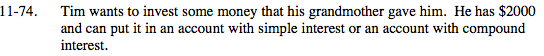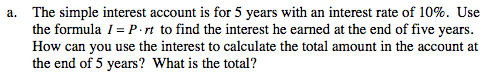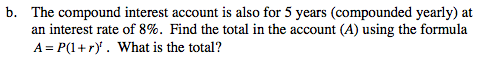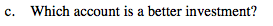### Home > MC2 > Chapter 11 > Lesson 11.2.4 > Problem11-74

11-74.Add the interest he earns to his initial principal to find the total amount he would have after 5 years.

I = ($2000)(.1)(5 years) I =$1000

Total = $2000 +$1000 = $3000A = 2000(1 + 0.8)5 (1.08)5 = 1.469 A =$2000 (1.469) = \$2938.66Which account has more money after 5 years?